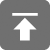# 工学1号馆

home

## [bx]和loop指令

Wu Yudong    September 13, 2016     Assembly   575

[bx]和内存单元的描述

（1）内存单元的地址；

（2）内存单元的长度（类型）。

[bx]同样也表示一个内存单元，它的偏移地址在bx中，比如下面的指令：

mov ax,[bx]

mov al,[bx]

（1）ax中的内容为0010H，我们可以这样来描述：(ax)=0010H；

（2）2000:1000 处的内容为0010H，我们可以这样来描述：(21000H)=0010H；

（3）对于mov ax,的功能，我们可以这样来描述：(ax)=((ds)*16+2)；

（4）对于mov ,ax 的功能，我们可以这样来描述：((ds)*16+2)=(ax)；

（7）对于push ax的功能，我们可以这样来描述：
(sp) = (sp)-2

((ss)*16＋(sp))=(ax)

mov ax,[idata]就代表mov ax,、mov ax,、mov ax,等。

mov bx,idata就代表mov bx,l、mov bx,2、mov bx,3等。

mov ds,idata就代表mov ds,1、mov ds,2等，它们都是非法指令。

## [BX]

mov ax,[bx]

mov [bx],ax

mov ax,2000H
mov ds,ax
mov bx,1000H
mov ax,[bx]
inc bx
inc bx
mov [bx],ax
inc bx
inc bx
mov [bx],ax
inc bx
mov [bx],al
inc bx
mov [bx],al

-r

AX=FFFF  BX=0000 CX=001D  DX=0000  SP=0000  BP=0000  SI=0000  DI=0000

DS=075A  ES=075A  SS=0769  CS=076A  IP=0000  NV  UP  EI  NZ  NA  PO  NC

076A:0000  B80020        MOV    AX,2000

-t

AX=2000  BX=0000 CX=001D  DX=0000  SP=0000  BP=0000  SI=0000  DI=0000

DS=075A  ES=075A  SS=0769  CS=076A  IP=0003  NV  UP  EI  NZ  NA  PO  NC

076A:0003  8ED8        MOV    DS,AX

-t

AX=2000  BX=0000 CX=001D  DX=0000  SP=0000  BP=0000  SI=0000  DI=0000

DS=2000  ES=075A  SS=0769  CS=076A  IP=0005  NV  UP  EI  NZ  NA  PO  NC

076A:0005  BB0010        MOV    BX,1000

-t

AX=2000  BX=1000 CX=001D  DX=0000  SP=0000  BP=0000  SI=0000  DI=0000

DS=2000  ES=075A  SS=0769  CS=076A  IP=0008  NV  UP  EI  NZ  NA  PO  NC

076A:0008  8B07        MOV    AX,[BX]                                            DS:1000=0000

ds=2000H，  bx=1000H；

-t

AX=0000  BX=1000 CX=001D  DX=0000  SP=0000  BP=0000  SI=0000  DI=0000

DS=2000  ES=075A  SS=0769  CS=076A  IP=000A  NV  UP  EI  NZ  NA  PO  NC

076A:000A   43            INC     BX

-t

AX=0000  BX=1001  CX=001D  DX=0000  SP=0000  BP=0000  SI=0000  DI=0000

DS=2000  ES=075A  SS=0769  CS=076A  IP=000B   NV  UP  EI  NZ  NA  PO  NC

076A:000B   43            INC     BX

-t

AX=0000  BX=1002  CX=001D  DX=0000  SP=0000  BP=0000  SI=0000  DI=0000

DS=2000  ES=075A  SS=0769  CS=076A  IP=000C   NV  UP  EI  NZ  NA  PO  NC

076A:000C   8907            MOV     [BX],AX                                                 DS:1002=0000

-t

AX=0000  BX=1004  CX=001D  DX=0000  SP=0000  BP=0000  SI=0000  DI=0000

DS=2000  ES=075A  SS=0769  CS=076A  IP=000E   NV  UP  EI  NZ  NA  PO  NC

076A:000E   43             INC     BX

-t

AX=0000  BX=1004  CX=001D  DX=0000  SP=0000  BP=0000  SI=0000  DI=0000

DS=2000  ES=075A  SS=0769  CS=076A  IP=0010   NV  UP  EI  NZ  NA  PO  NC

076A:0010   8907            MOV     [BX],AX                                                 DS:1004=0000

-t

AX=0000  BX=1004  CX=001D  DX=0000  SP=0000  BP=0000  SI=0000  DI=0000

DS=2000  ES=075A  SS=0769  CS=076A  IP=0012   NV  UP  EI  NZ  NA  PO  NC

076A:0012   43                  INC     BX

-t

AX=0000  BX=1005  CX=001D  DX=0000  SP=0000  BP=0000  SI=0000  DI=0000

DS=2000  ES=075A  SS=0769  CS=076A  IP=0013   NV  UP  EI  NZ  NA  PO  NC

076A:0013   8807            MOV     [BX],AL                                                 DS:1005=00

## LOOP指令

① (cx)=(cx)-1；

② 判断cx中的值，不为零则转至标号处执行程序，如果为零则向下执行。

(ax)= (ax)*2*2*2*2*2*2*2*2*2*2*2，最后(ax)中为2∧12的值。N*2可用N+N 实现。

assume cs:code
code segment
mov ax,2
mov cx,11
loop s
mov ax,4c00h
int 21h
code ends
end

（1）标号

（2）loop s

CPU 执行loop s的时候，要进行两步操作：

① (cx)=(cx)-1；

② 判断cx 中的值，不为0 则转至标号s 所标识的地址处执行(这里的指令是“add ax,ax)，如果为零则执行下一条指令(下一条指令是mov ax,4c00h)。

（3）以下三条指令

mov cx,11

s: ddd ax,ax

loop s